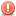CodeGuru Home VC++ / MFC / C++ .NET / C# Visual Basic VB Forums Developer.com

# Thread: Converting C++ to Java

1.Junior MemberJoin Date
Apr 2018
Posts
1

##Converting C++ to Java

Hello I need help converting this C++ code to java. I have tried myself, but was not able to understand how to be able to do that. Here is the code, and I'm sorry if you are just as stuck as I am. Thank you!

Code:

Code:
```class Student implements Comparable<Student> {
String name;
String Id;
int score;

// ----------------------------------------------------------------------------------
// Student constructor
// ----------------------------------------------------------------------------------
public Student(String studentName, String studentId, int studentScore) {
name = studentName;
Id = studentId;
score = studentScore;
}

public Student() {

name = "";
Id = "";
score = -1;

}

// ----------------------------------------------------------------------------------
// Setters for the attributes of a student
// ----------------------------------------------------------------------------------
void setName(String studentName) {

name = studentName;
}

void setId(String studentId) {
Id = studentId;
}

void setScore(int studentScore) {
score = studentScore;
}
// ----------------------------------------------------------------------------------

// Getters for the attributes of a student
// ----------------------------------------------------------------------------------

String getName() {
return name;
}

String getId() {
return Id;

}

int getScore() {
return score;
}

// -----------------------------------------------------------------
// Create a one-line description of the student as a string
// -----------------------------------------------------------------
public String toString() {
String studentInfo = score + "\t\t" + Id + "\t\t" + name;
return (studentInfo);
}

@Override
public int compareTo(Student aStudent) {
if (this.score < aStudent.score)
return -1;
else if (this.score == aStudent.score)
return 0;
else
return 1;

}
}

import java.util.Scanner;

public class StudentRoster {

static Scanner scan = new Scanner(System.in);

// Declare the roster of size ARRAY_MAX_SIZE below.
static int SIZE = 10;

// Get the student information from the keyboard
private static Student getStudentInfo() {
System.out.print("Enter student name : ");
String name = scan.nextLine();
System.out.print("Enter student id : ");
String id = scan.nextLine();
System.out.print("Enter student score : ");
int score = Integer.parseInt(scan.nextLine());

Student std = new Student(name, id, score);
return std;
}

// Display output heading
private static void displayHeading() {
System.out.println("\n----- STUDENT ROSTER -----\n");
System.out.println("***********************************************************************");
System.out.println("By John Doe, CS1301-Section xx, Spring 2017");
System.out.println("***********************************************************************\n");
}

// Display the menu
private static void displayMenu() {
System.out.print(
"\nAvailable operations:\n1. Add a student\n2. Delete a student\n3. Display at and above the average students\n4. Display all the students\n9. End the program\n\nEnter your desired option: ");
}

// find the student by his id
private static int findStudent(Student[] roster, int numofstudents, String studentId) {
int result = -1;
for (int i = 0; i < numofstudents; i++) {
if (roster[i].Id.equals(studentId))
return i;
}
return (result);
}

// Compute the average score of all the students
private static double average(Student[] roster, int numofstudents) {
double total = 0;
for (int i = 0; i < numofstudents; i++) {
total += roster[i].score;
}
return total / numofstudents;
}

public static void main(String[] args) {

final int SENTINEL = 9;
Scanner sc = new Scanner(System.in);
Student roster[] = new Student[SIZE];
int numofstudents = 0;
int input;
displayHeading();
while (true) {
displayMenu();
input = Integer.parseInt(sc.nextLine());
switch (input) {
case 1:
roster[numofstudents++] = getStudentInfo();
break;

case 2:
if (numofstudents <= 0)
System.out.println("Sorry, there are no students to delete!");
else {
System.out.print("Enter the student Id: ");
String id = sc.nextLine();
int index = findStudent(roster, numofstudents, id);
if (index == -1)
System.out.println("The student with Id " + id + " is not found.");
else
for (int i = index; i < numofstudents - 1; i++)
roster[i] = roster[i + 1];

numofstudents--;
}
break;

case 3:
if (numofstudents <= 0)
System.out.println("Sorry, there are no students to display!");
else {
System.out.println("Students are :");
for (int i = 0; i < numofstudents; i++) {
if (roster[i].score >= average(roster, numofstudents)) {
System.out.println(roster[i]);
}
}
}
break;

case 4:
if (numofstudents <= 0)
System.out.println("Sorry, there are no students to display!");
else {
System.out.println("Students are :");
for (int i = 0; i < numofstudents; i++) {

System.out.println(roster[i]);

}
}
break;

case 9:
break;
}

if (input == 9)
break;
}
System.out.println("\nHave a nice day!\n");

}

}```
Last edited by 2kaud; April 26th, 2018 at 08:46 AM. Reason: Added code tagsReply With Quote

2.MemberJoin Date
Feb 2017
Posts
429

## Re: Converting C++ to JavaOriginally Posted by MissPianist43I need help converting this C++ code to java.
You don't need to because it is Java already.Reply With Quote

3.Junior MemberJoin Date
May 2017
Posts
3

## Re: Converting C++ to Java

Did you mean converting it from Java to C++?Reply With Quote

####Posting Permissions

• You may not post new threads
• You may not post replies
• You may not post attachments
• You may not edit your posts
•Click Here to Expand Forum to Full Width

On-Demand Webinars (sponsored)